Question

# 10-3 Hillside issues \$2,100,000 of 5%, 15-year bonds dated January 1, 2017, that pay interest semiannually...

10-3

Hillside issues \$2,100,000 of 5%, 15-year bonds dated January 1, 2017, that pay interest semiannually on June 30 and December 31. The bonds are issued at a price of \$2,570,390.

Required:

1. Prepare the January 1, 2017, journal entry to record the bonds’ issuance.
2(a) For each semiannual period, complete the table below to calculate the cash payment.
2(b) For each semiannual period, complete the table below to calculate the straight-line premium amortization.
2(c) For each semiannual period, complete the table below to calculate the bond interest expense.
3. Complete the below table to calculate the total bond interest expense to be recognized over the bonds' life.
4. Prepare the first two years of an amortization table using the straight-line method
5. Prepare the journal entries to record the first two interest payments.

For each semiannual period, complete the table below to calculate the cash payment, straight-line premium amortization and bond interest expense. (Round "Unamortized Premium" to whole dollar and use the rounded value for part 4 & 5.)

 Par (maturity) value Annual Rate Year Semiannual cash interest payment not attempted not attempted not attempted not attempted not attempted = not attempted Bond price Par (maturity value) Premium on Bonds Payable Semiannual periods Straight-line premium amortization not attempted not attempted not attempted = not attempted not attempted not attempted = not attempted Semiannual cash payment Premium amortization Bond interest expense not attempted not attempted not attempted = not attempted

 Journal entry Date General Journal Debit Credit 1/1/2018 Cash 2,570,390 premium on bonds 470,390 bonds payable 2,100,000 2-a) par maturity value Annual rate / year semi annual cash payment 2,100,000 * 5% 6./12 52500 semi annual Straight line 2-b) bond price par value premium periods premium amortization 2,570,390 - 2,100,000 = 470,390 / 30 = 15680 2-c) Semi annual cash premium bond interest expense payment amortization 52,500 - 15680 = 36,820 3) total bond interest expense over life of bonds amount repaid 30 payments of 52,500 1575000 par value ant maturity 2,100,000 total repaid 3675000 less amount borrowed 2,570,390 total bond interest expense. 1,104,610 (note bond interest expense may differ slightly due to rounding) 4) unamort Carrying period premium value 1/1/2018 470,390 2,570,390 06/30/18 454,710 2,554,710 12/31/18 439,031 2,539,031 06/30/19 423,351 2,523,351 12/31/19 407,671 2,507,671 5) Date General Journal Debit Credit 6/30/18 interest expense 36,820 premium on bonds payable 15,680 cash 52,500 31/12/2018 interest expense 36,820 premium on bonds payable 15,680 cash 52,500

#### Earn Coins

Coins can be redeemed for fabulous gifts.

Similar Homework Help Questions
• ### Hillside issues \$2,100,000 of 5%, 15-year bonds dated January 1, 2017, that pay interest semiannually on...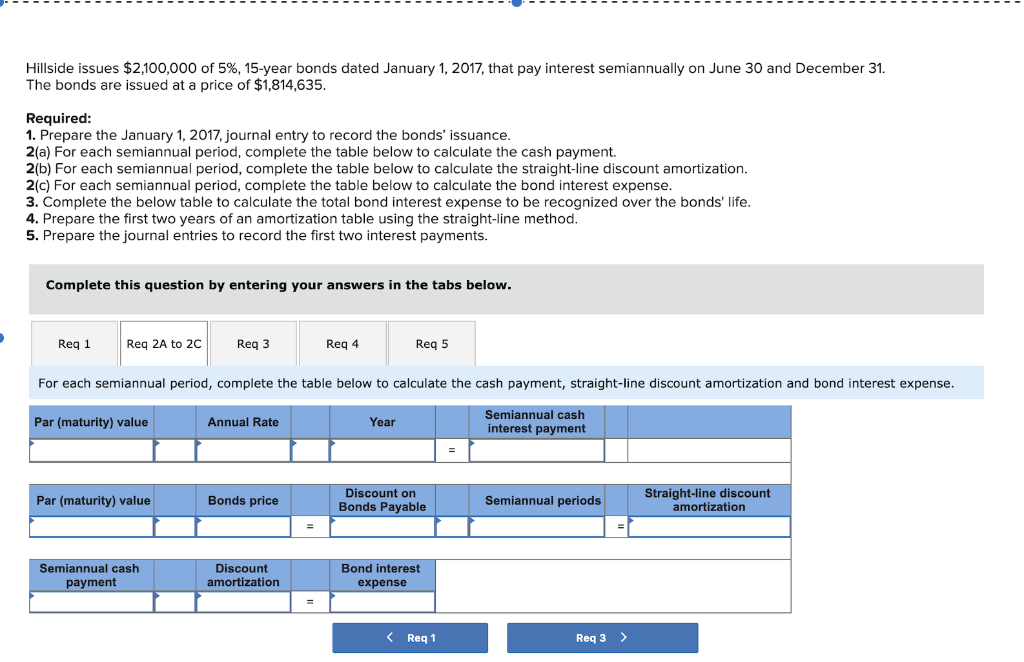Hillside issues \$2,100,000 of 5%, 15-year bonds dated January 1, 2017, that pay interest semiannually on June 30 and December 31 The bonds are issued at a price of \$1,814,635. Required: 1. Prepare the January 1, 2017, journal entry to record the bonds' issuance. 2(a) For each semiannual period, complete the table below to calculate the cash payment 2(b) For each semiannual period, complete the table below to calculate the straight-line discount amortization 2(c) For each semiannual period, complete the...

• ### Hillside issues \$4,000,000 of 6%, 15-year bonds dated January 1, 2017, that pay interest semiannually on...

Hillside issues \$4,000,000 of 6%, 15-year bonds dated January 1, 2017, that pay interest semiannually on June 30 and December 31. The bonds are issued at a price of \$4,895,980. Required: 1. Prepare the January 1, 2017, journal entry to record the bonds’ issuance. 2(a) For each semiannual period, complete the table below to calculate the cash payment. 2(b) For each semiannual period, complete the table below to calculate the straight-line premium amortization. 2(c) For each semiannual period, complete the...

• ### Hillside issues \$2,900,000 of 9%, 15-year bonds dated January 1, 2017 that pay interest semiannually on...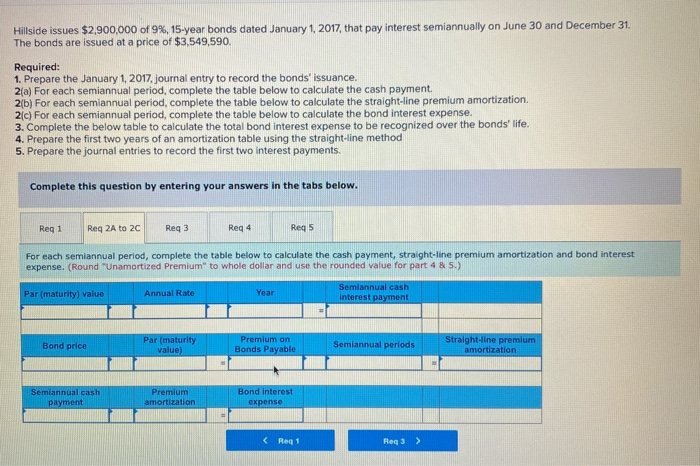Hillside issues \$2,900,000 of 9%, 15-year bonds dated January 1, 2017 that pay interest semiannually on June 30 and December 31 The bonds are issued at a price of \$3,549,590. Required: 1. Prepare the January 1, 2017, journal entry to record the bonds' issuance. 2(a) For each semiannual period, complete the table below to calculate the cash payment. 2(b) For each semiannual period, complete the table below to calculate the straight-line premium amortization. 2(c) For each semiannual period, complete the...

• ### Hillside issues \$2,100,000 of 5%, 15-year bonds dated January 1, 2017, that pay interest semiannually on...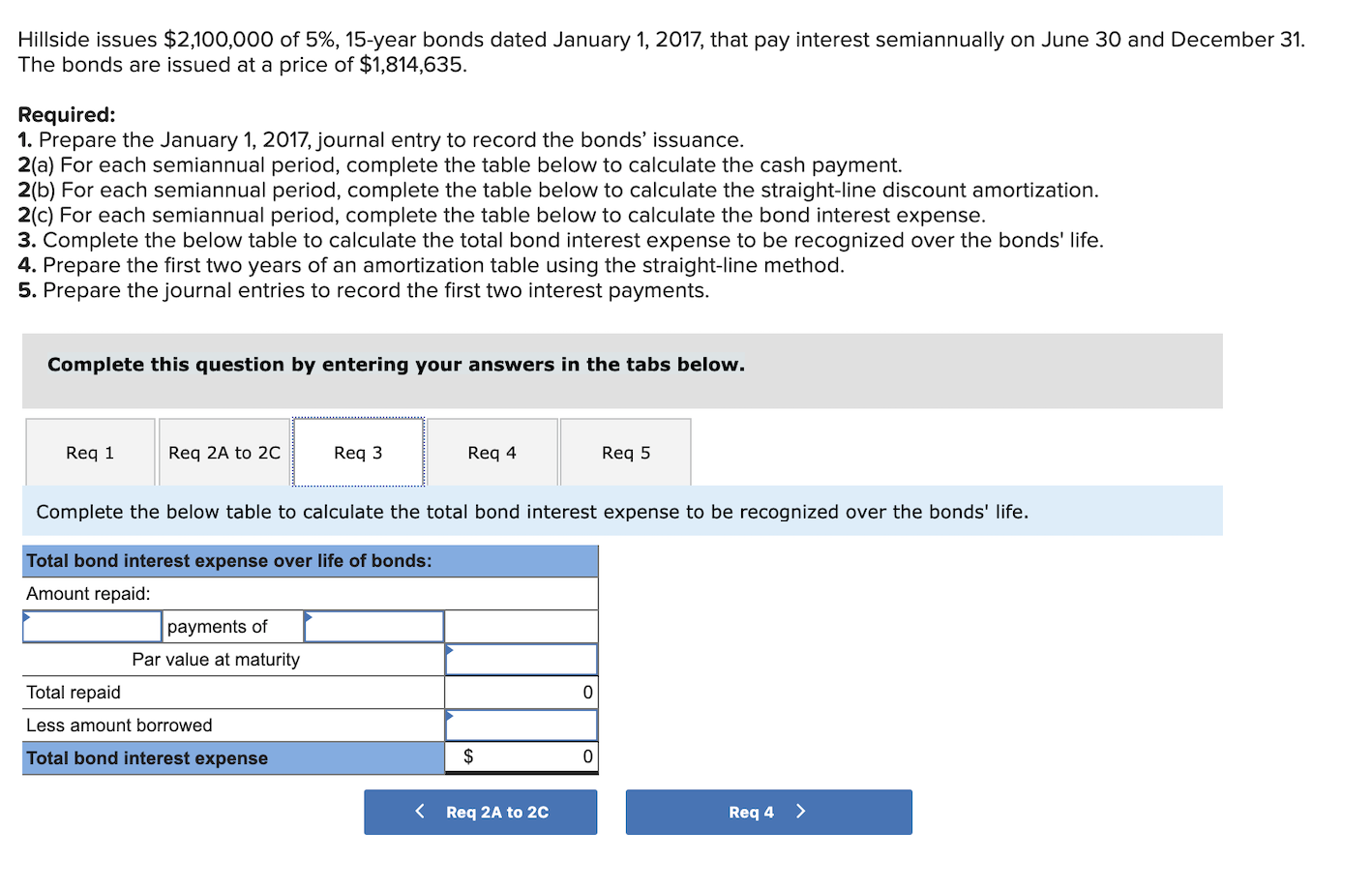Hillside issues \$2,100,000 of 5%, 15-year bonds dated January 1, 2017, that pay interest semiannually on June 30 and December 31. The bonds are issued at a price of \$1,814,635. Required: 1. Prepare the January 1, 2017, journal entry to record the bonds' issuance. 2(a) For each semiannual period, complete the table below to calculate the cash payment. 2(b) For each semiannual period, complete the table below to calculate the straight-line discount amortization. 2(c) For each semiannual period, complete the...

• ### Hillside issues \$2,600,000 of 5%, 15-year bonds dated January 1, 2017, that pay interest semiannually on...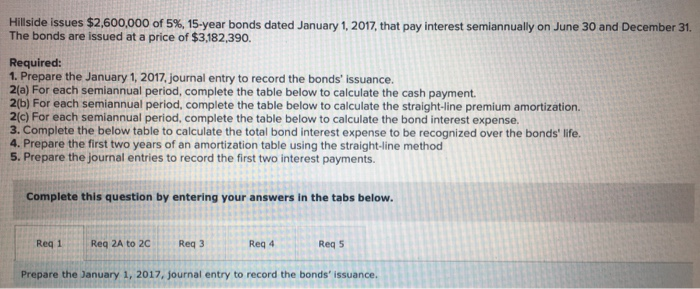Hillside issues \$2,600,000 of 5%, 15-year bonds dated January 1, 2017, that pay interest semiannually on June 30 and December 31 The bonds are issued at a price of \$3,182,390. Required: 1. Prepare the January 1, 2017, journal entry to record the bonds' issuance. 2(a) For each semiannual period, complete the table below to calculate the cash payment. 2(b) For each semiannual period, complete the table below to calculate the straight-line premium amortization. 2(C) For each semiannual period, complete the...

• ### Hillside issues \$2,100,000 of 5%, 15-year bonds dated January 1, 2017, that pay interest semiannually on...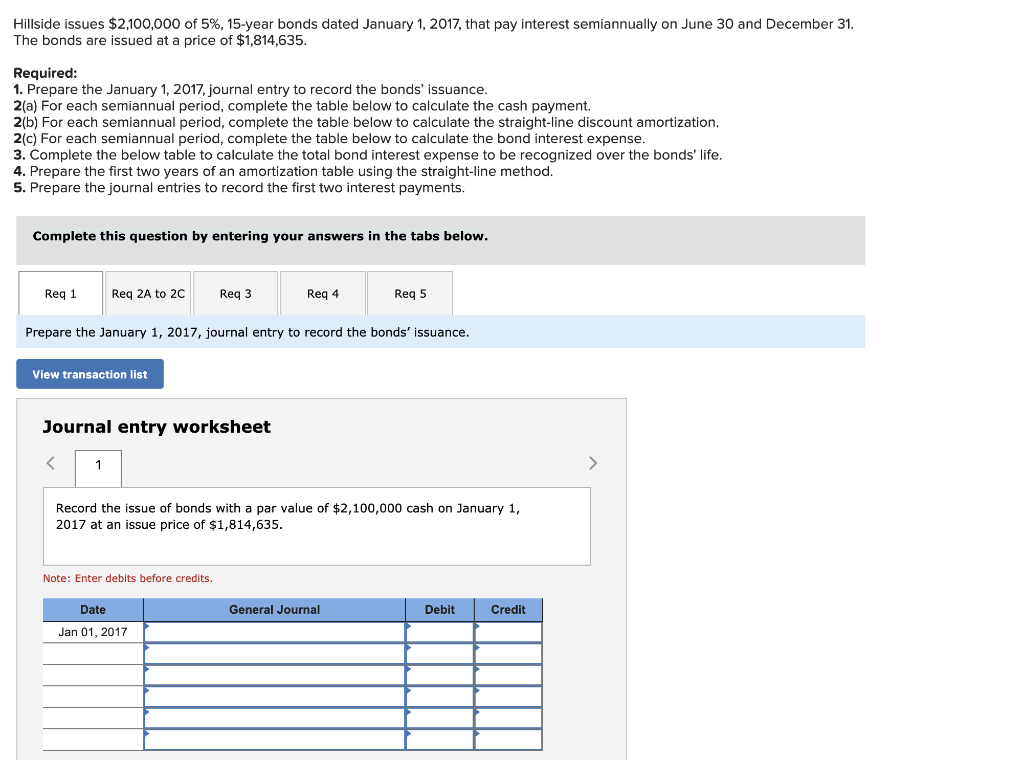Hillside issues \$2,100,000 of 5%, 15-year bonds dated January 1, 2017, that pay interest semiannually on June 30 and December 31. The bonds are issued at a price of \$1,814,635. Required: 1. Prepare the January 1, 2017, journal entry to record the bonds' issuance. 2(a) For each semiannual period, complete the table below to calculate the cash payment. 2(b) For each semiannual period, complete the table below to calculate the straight-line discount amortization 2(c) For each semiannual period, complete the...

• ### Hillside issues \$4,000,000 of 6% , 15-year bonds dated January 1, 2017, that pay interest semiannually...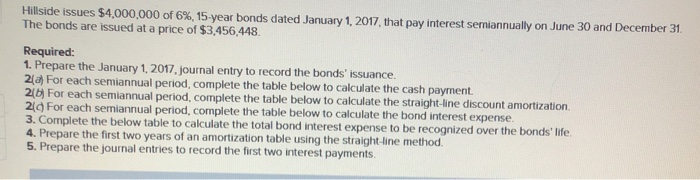Hillside issues \$4,000,000 of 6% , 15-year bonds dated January 1, 2017, that pay interest semiannually on June 30 and December 31 The bonds are issued at a price of \$3,456,448. Required: 1. Prepare the January 1, 2017, journal entry to record the bonds' issuance. 2d For each semiannual period, complete the table below to calculate the cash payment. 2( For each semiannual period, complete the table below to calculate the straight-line discount amortization. 20 For each semiannual period, complete...

• ### Hillside issues \$1,500,000 of 6%, 15-year bonds dated January 1, 2017, that pay interest semiannually on June 3...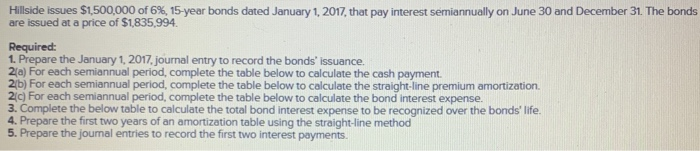Hillside issues \$1,500,000 of 6%, 15-year bonds dated January 1, 2017, that pay interest semiannually on June 30 and December 31. The bonds are issued at a price of \$1,835,994. Required: 1. Prepare the January 1, 2017, journal entry to record the bonds' issuance. 21a) For each semiannual period, complete the table below to calculate the cash payment. 2b) For each semiannual period, complete the table below to calculate the straight-line premium amortization 21c) For each semiannual period, complete the...

• ### Hillside issues \$2,600,000 of 5%, 15-year bonds dated January 1, 2017, that pay interest semiannually on...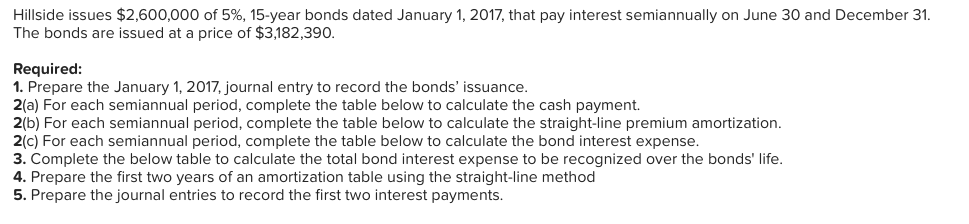Hillside issues \$2,600,000 of 5%, 15-year bonds dated January 1, 2017, that pay interest semiannually on June 30 and December 31. The bonds are issued at a price of \$3,182,390. Required: 1. Prepare the January 1, 2017, journal entry to record the bonds' issuance. 2(a) For each semiannual period, complete the table below to calculate the cash payment. 2(b) For each semiannual period, complete the table below to calculate the straight-line premium amortization. 2(c) For each semiannual period, complete the...

• ### Hillside issues \$2,600,000 of 5%, 15-year bonds dated January 1, 2017, that pay interest semiannually on...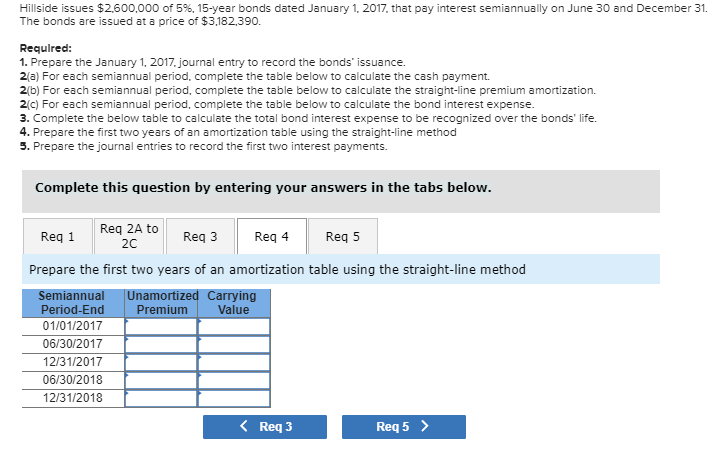Hillside issues \$2,600,000 of 5%, 15-year bonds dated January 1, 2017, that pay interest semiannually on June 30 and December 31. The bonds are issued at a price of \$3,182,390 Required: 1. Prepare the January 1, 2017, journal entry to record the bonds' issuance. 2a) For each semiannual period, complete the table below to calculate the cash payment 2(b) For each semiannual period, complete the table below to calculate the straight-line premium amortization. 2c) For each semiannual period, complete the...